#### You may also like### I'm Eight

Find a great variety of ways of asking questions which make 8.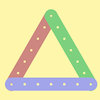### Let's Investigate Triangles

Vincent and Tara are making triangles with the class construction set. They have a pile of strips of different lengths. How many different triangles can they make?### Noah

Noah saw 12 legs walk by into the Ark. How many creatures did he see?

# Making Longer, Making Shorter

##### Age 5 to 7Challenge Level

First, Ahmed used interlocking cubes to make a rod four cubes long: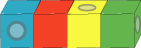How many cubes did he need to make a rod twice the length of that one?
How many cubes did he need to make one three times the length?
How many cubes did he need to make one four times the length?
How many cubes did he need to make a rod half the length of his first one?
How many cubes did he need to make a rod a quarter of the length of his first one?

These rods are the ones Ahmed made: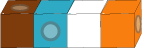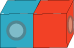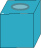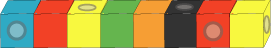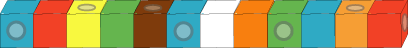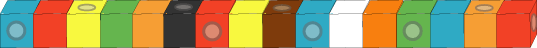Which one is twice the length of Ahmed's first rod?
Which one is three times the length?
Which one is four times the length?
Which one is half the length of his first rod?
Which one is a quarter of the length of his first rod?
Which one is the same length as his first rod?